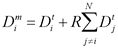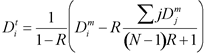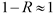Spitzer Documentation & Tools
IRS Instrument Handbook

### 5.1.6             DROOPOP

Droop causes all samples across the array to have a larger signal value than they should. This phenomenon applies only to IRS SUR data, and not to Double Correlated Sampling (DCS) data (the data mode used during IRS Peak-Up Acquisition).  Since we are measuring the accumulation of (negative) charge, ramps that become more negative appear to “droop”, hence the origin of the term. The total charge on the array contributes to the droop for each pixel. A theoretical model of the droop effect does not exist. An empirical model indicates that an observed sample value, Dmi (ith pixel in the array of N elements, N being 16384 for IRS), and the same sample value in the absence of the effect, Dti,  are related by a so-called droop coupling constant R and the sum of remaining sample values in the array:Equation 5.6

The above empirical relation applies to each plane of a 3-dimensional DCE. The relation can be algebraically manipulated to show that the droop effect can be corrected by subtracting a nearly constant offset from all samples in each plane of the DCE. The offset is unique to each plane of the DCE:Equation 5.7

In the IRS pipelines, the droop coupling constant R is assumed to be the same for all pixels. Each IRS instrument channel has its own droop coupling constant. In the IRS pipelines, the input droop “coefficient” is actually the quantity (N-1)R, and it is 0.45, 0.49, 0.20, and 0.19 for SL, SH, LL, and LH, respectively, with an uncertainty of 15%. Therefore,since N is very large.

The algorithm for correcting for droop thus consists of these steps:

-Sum up all samples in a given DCE plane.

-Subtract a constant offset from all samples of the plane, which depends on the input droop coefficient and the sum of all samples of the plane.

-Re-scale samples by 1/(1-R), although the factor is nearly unity (to within 10−5).

In the IRS pipelines, the input to DROOPOP (“Droop Operation” or correction) is the output of SATCOR (satcor.fits), where saturated input samples were saturation-corrected. The purpose of using saturation-corrected samples is to estimate, as accurately as possible, the sum of signal in the entire array. This is because droop occurs in either the detectors or multiplexer, before A/D conversion. After the execution of DROOPOP per the above algorithm, the samples that were saturation-corrected by SATCOR are reset to a constant arbitrary value (300,000 electrons). The particular value has no consequence since it is not used by any subsequent pipeline module.

The outputs of this module are droopop.fits and droopop_unc.fits. These files are not available in the Spitzer Heritage Archive, but can be generated by running CUPID.